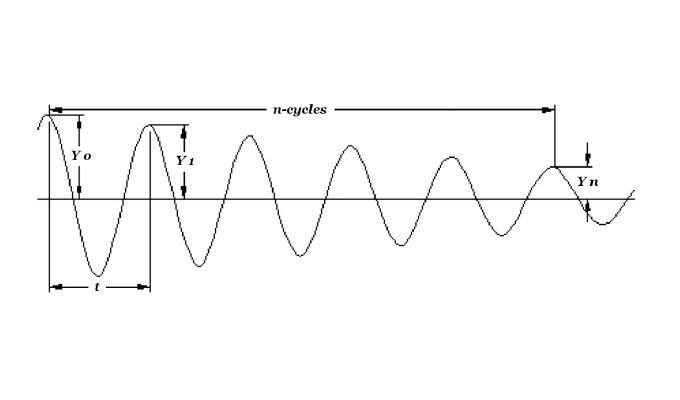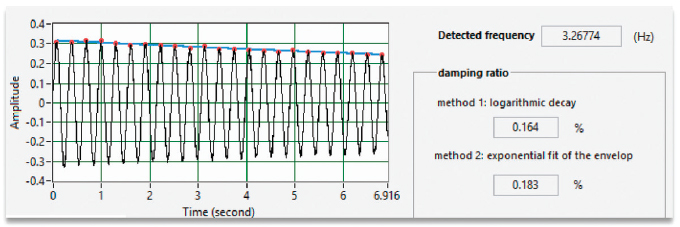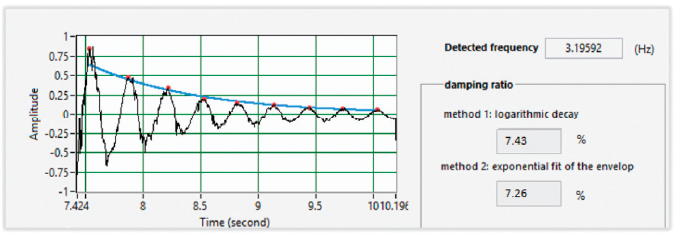By Dr. Budhaditya Hazra, Liang Xu, and Dr. Suri Ganeriwala, SpectraQuest

In last month’s introduction, we touched on how SpectraQuest has introduced a new innovative and modular bench scale experimental set-up called vibration fundamentals training system. The Vibration Fundamentals Training System (alias VFT) is a turn-key integrated educational package for teaching/learning the fundamental principles of mechanical vibration as well as engineering mechanics. The VFT provides an ideal tool for damping free vibration experiments using the wireless sensors without affected by the damping associated with the sensor cables. It is a perfect tool for teaching mechanical vibration courses both at under graduate and graduate levels.

### EXPERIMENT 2: FREE VIBRATION OF SPRING MASS DAMPER SYSTEM

In general, vibratory systems consist basically of potential energy storing element (stiffness), kinetic energy storing element (mass or inertia), and energy dissipation element (damping). Damping effect in vibratory systems may be caused by friction between moving parts (dry friction), or material deformation and internal friction between layers of a part (structural action), or the use of mechanical viscous dampers. The first two may never be completely eliminated but can be, generally, ignored under certain conditions.

However, the third can be used to achieve a required damping effect. In the first part of this series, we introduced some experiments that can be easily performed as a part of the vibration teaching curriculum, and we conclude with experiments showing that the damping contribution from dry friction and structural action is indeed negligible.

### OBJECTIVES

In this experiment, a simple spring-mass-damper system is to be studied, in order to determine the damping coefficient C by logarithmic decrement method.

### SYSTEM DESCRIPTION

Figure 4 shows the system to be studied, which consists of a solid disc of mass M (sum of the disc mass, springs effective mass and the load career), that moves vertically up and down, while attached from its upper side by a spring of stiffness K, and from its lower side by a dashpot damper with damping coefficient C; the spring and the damper are fixed to the main frame.

The dashpot used consists of a cylindrical disk immersed in a container filled with oil. Figure 6 shows the details of the cylinder piston type arrangement. The coefficient of damping of the dashpot varies according to the spacing between the disc and the wall of the container. All the calculations are performed based on the acceleration measurement carried out using the wireless accelerometer.

### GOVERNING EQUATIONS

By giving the system shown schematically in figure 4 an initial vertical displacement Y, it will vibrate freely with a time-varying function y(t), and the resulting equation of motion will be:

To solve for y(t); let y(t) = Yest, then the auxiliary equation and its solutions are:

But:

Then, the linear spring equation following Hooke’s law becomes:

### LOGARITHMIC DECREMENT METHOD

Considering a typical decaying curve as the one shown in figure 5, then the ratio of the amplitude Yo corresponding to the time t = to, to the amplitude Yn at time t = to + nT, is given by:

Define the logarithmic decrement as:

Eliminate a portion of the logarithmic decrement from the previous two equations to obtain the following:

Then find the damping coefficient, where:

### THEORETICAL VALUES OF DAMPING RATIO

The theoretical expression for viscous damping coefficient for the piston cylinder arrangement as shown in figure 6 is given by the following formula

Where u is the coefficient of kinematic viscosity in micro-reyns (or centistokes). The damping coefficient is expressed in Ns / m2. Once c is known, the theoretical value of damping ratio can be calculated using the following expression:

To arrive at the theoretical values of damping ratio using equation the following values of the parameters are used: l = 6.5 mm; d = 4.15 mm; = 3 micro-reyns. The diameter of the cylindrical cup containing the damping fluid is 73 mm.

### EXPERIMENTAL PROCEDURES

2. Pull the mass down and then release it to let it oscillate freely.
3. The accelerometer gathers the acceleration data.
4. Use the software to calculate natural frequency and damping using Fourier spectra and logarithmic decrement respectively.
5. Perform two sets of experiments for two situations: with the damper attached and no damper attached.

From the results in tables 3 and 4, it can be observed that even the undamped system possesses some damping although negligibly small. The theoretical value of damping ratio is calculated. It can be observed that there is significant error between the actual and the estimated values of damping. This is attributed to the fact that there are many unobservable sources of damping which is not practically easy to determine. For example, the movement of the cylindrical rod that connects the vibration mass to the piston, generates friction between the rod and the guide through which it slides. Figures 7 and 8 further provides the screen capture for the damping and frequency estimates of the spring-mass-damper system using the customized VFT software.

Thus, it is safe to conclude that accurate estimation of damping is practically difficult. The problem gets mitigated to a large extent by increasing the mass of the vibrating body keeping the stiffness fixed.Figure 7: Estimated wn and the theoretical values of damping ratio using the custom software for the undamped case.Figure 8: Estimated wn and the theoretical values of damping ratio using the custom software for the damped case.

### VIRTUAL EXPERIMENTATION

One of the key aspects of the VFT system is virtual experimentation. The key philosophy behind virtual experimentation is that the students should be able to perform all the experiments on a simulation setting before performing the actual experiment. The VFT package offers a simulation software is designed to teach basic concepts using a new interactive and visual simulation technique. Users can perform virtual experiments on various topics by changing the parameters of a vibratory system and see how the system behavior is affected. The effect is displayed dynamically. The vivid visualization enhances the learning and clarification. A spring-mass-damper system is used to animate the vibration response. It is important to observe that the speed of animation is proportional to the natural frequency of the spring mass system: the higher the frequency, the faster it moves.

One of the attractive features of the software is the multi-plot option. A multi-plot can be used to compare their effects on vibration response. Therefore, instead of deriving the equations, the user can go one step further, “play and see” the vibration behavior. The software includes the most common topics of a typical vibration course. This is particularly u seful, for example, to demonstrate resonance of a SDOF vibrating system. Users can set the frequency of the forcing function close to the natural frequency of the SDOF system and compare the response amplitudes as the forcing function frequency is perturbed to values slightly higher and lower than the natural frequency.

### CONCLUSION

We have presented a very innovative Vibration Learning System; SpectraQuest’s vibration fundamental training (VFT) system. VFT is a modular, attractive, hands on vibration training system that supplements textbook learning with very easy, convenient and effective bench scale experiments. The system facilitates different levels of modularization to perform wide gamut of vibration experiments. The results from some of the experiments clearly prove this point. It is well supplemented by a wonderful virtual experimental package in the form of a simulation software which clearly demonstrates its efficacy in providing a preparatory guidance before performing the actual laboratory tests.